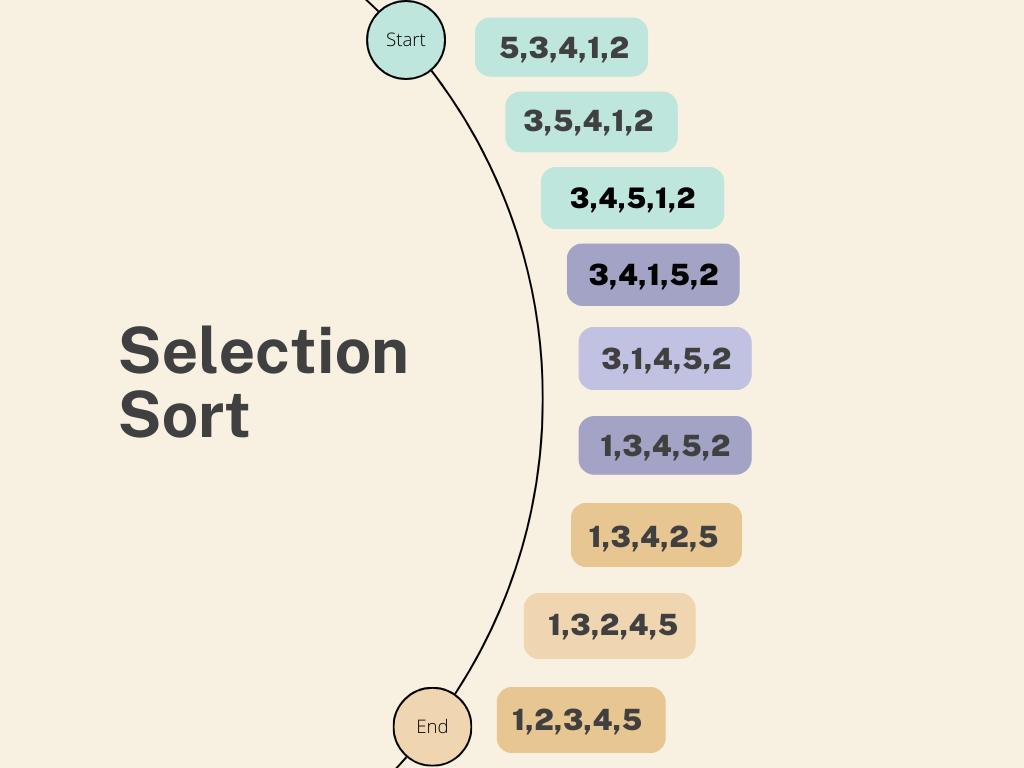# Selection-Sort Algorithm

Selection sort is an in-place comparison sorting algorithm. It divides the input array into two parts: the sorted subarray at the beginning and the unsorted subarray at the end. The algorithm repeatedly selects the minimum (or maximum) element from the unsorted subarray and swaps it with the first unsorted element, expanding the sorted subarray by one element.

## Explanation

A brief explanation of the selection sort algorithm:

• Selection sort is an in-place comparison sorting algorithm: This means that selection sort does not require additional memory beyond the input array, and elements are compared to determine their relative order.

• It divides the input array into two parts: Conceptually, selection sort divides the array into two subarrays: the sorted subarray at the beginning and the unsorted subarray at the end. Initially, the sorted subarray is empty, and the unsorted subarray contains all the elements.

• The algorithm repeatedly selects the minimum (or maximum) element: In each iteration, selection sort identifies the minimum (or maximum) element from the unsorted subarray. The selection process involves scanning the unsorted subarray to find the smallest (or largest) element.

• It swaps the selected element with the first unsorted element: Once the minimum (or maximum) element is identified, selection sort swaps it with the first element of the unsorted subarray. This action effectively expands the sorted subarray by one element and reduces the unsorted subarray by one.

• The process continues until the entire array is sorted: Selection sort repeats the selection and swapping steps for the remaining unsorted elements until the entire array becomes sorted. The sorted subarray gradually grows, and the unsorted subarray shrinks until no elements remain in the unsorted portion.

• Selection sort is a sorting algorithm that repeatedly finds the minimum element in the unsorted portion of an array and swaps it with the element at the beginning of the unsorted section. This process continues until the entire array is sorted. The algorithm has a time complexity of O(n^2), where n is the number of elements in the array. It performs n iterations, each involving a comparison of each element in the unsorted section with the current minimum. As a result, the total number of comparisons is roughly (n * (n-1))/2. While selection sort is inefficient for large input sizes, it can be useful for small arrays or when minimizing the number of swaps is a priority.

Graphical representation of Selection Sort:## Example

The following example written in Java shows an implementation of selection sort and then uses it to sort `{5, 3, 4, 1, 2}`:

```public class SelectionSort {    public static void selectionSort(int[] array) {        int n = array.length;        for (int i = 0; i < n - 1; i++) {            int minIndex = i;            for (int j = i + 1; j < n; j++) {                if (array[j] < array[minIndex]) {                    minIndex = j;                }            }            int temp = array[minIndex];            array[minIndex] = array[i];            array[i] = temp;        }    }
public static void main(String[] args) {        int[] array = {5, 3, 4, 1, 2};        selectionSort(array);        System.out.println("Sorted array: ");        for (int i : array) {            System.out.print(i + " ");        }    }}
```

The output for the above code is:

```Sorted array: 1 2 3 4 5
```

The `selectionSort` function in the code takes an integer `array` as input and initializes a variable `n` with the length of the array. The outer loop iterates through the elements from the first to the second-to-last element of the array, representing the boundary of the unsorted subarray.

Inside the outer loop, an inner loop starts from `i+1` and searches for the minimum element in the unsorted portion of the array. The index of the smallest element found is stored in the `minIndex` variable.

After the inner loop completes, the algorithm swaps the found minimum element with the first element of the unsorted subarray using a temporary variable (`temp`). This ensures that the minimum element is correctly positioned in the sorted subarray.